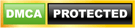# Different ways to create a table and insert data into the table

## Introduction

Tables can be defined as the structure which contains the data in the database. This article discuss about the different ways to create a table and insert data into the table. Some of the ways to create a table is given below:-

### 1)Creation of a table with the help of a create statement

For example, suppose we want to create a table called tbl_student then the syntax of creating this table is given below:-

```CREATE TABLE tbl_Students( [Studentid] [int] IDENTITY(1,1) NOT NULL, [Firstname] [nvarchar](200) , [Lastname] [nvarchar](200) , [Email] [nvarchar](100) )```

Let us suppose we want to insert data into the table tbl_students, then we can use the insert statements to insert data into the table.

The insert statement used for inserting the data is given below:-

```Insert into tbl_students(Firstname,lastname,email) select 'Vivek','Johari','Vivek@abc.com' union all select 'Pankaj','Kumar','Pankaj@abc.com' union all Select 'Amit','Singh','amit@abc.com'```

Here we can insert multiple rows into the table with the help of a single query mentioned above.

Another way to insert the values into the table tbl_students is :-

```Insert into tbl_students(Firstname,lastname,email) Values('Manish','Kumar','manish@abc.com')```

```Insert into tbl_students(Firstname,lastname,email) Values('Abhishek','Singh','abhishek@abc.com')```

```Insert into tbl_students(Firstname,lastname,email) Values ('Uma','Sharma','Uma@abc.com')```

Here, whenever we need to insert a row into the table ,we have to use a seperate insert statement.

The result of the above Sql queries can be verified with the help of the following query:-

`SELECT * FROM tbl_students`

Result:-

### Note:-

We don’t need to insert data into the column Studentid since it is defined as the Identity column and whenever a record is inserted into the table, sql server automatically insert value into this column.

### 2)Creation of table with the help of another table.

2.1) Suppose we want to create a table tbl_studentinfo which contains a subset of the columns (studentid, Firstname, Lastname) of the table tbl_student then we can use the following query.

`Select studentid, Firstname, Lastname into tbl_studentinfo from tbl_students`

This query will create a table tbl_studentinfo having columns studentid, Firstname, Lastname and it contains data of these columns from the table tbl_students. This can be verify with the help of the given below query.

`SELECT * FROM tbl_studentinfo`

Result:-

2.2)Suppose we want to create a table which is exactly the copy of a given table then we can also use the following Sql query

`SELECT * INTO tbl_studentscopy FROM tbl_students`

The table tbl_studentscopy created by the above Sql query will contains exactly the same data as tbl_students. This can be verified with the help of the query given below:-

`SELECT * FROM tbl_studentscopy`

Result:-

There is another interesting way to insert the values into a table with the help of another table. For example, suppose we have a table named as tbl_Studentsdemo whose structure is given below:-

```CREATE TABLE tbl_Studentsdemo( [Studentid] [int] IDENTITY(1,1) NOT NULL, [Firstname] [nvarchar](200) , [Lastname] [nvarchar](200) , [Email] [nvarchar](100) )```
Now if we want to insert values into the table from the table tbl_students, then we can use the following sql query to insert the data into the table.

```Insert into tbl_Studentsdemo(Firstname,lastname,email) SELECT Firstname,lastname,email FROM tbl_students```

The above query will insert all the data from the table tbl_students into the table tbl_Studentsdemo. Again we can verified it with the help of the given query:-

`SELECT * FROM tbl_Studentsdemo`

Result:-## 1 thought on “Different ways to create a table and insert data into the table”

1.gaur arun says:

i want more way except these 2 ways (using create or copy a table)